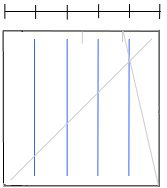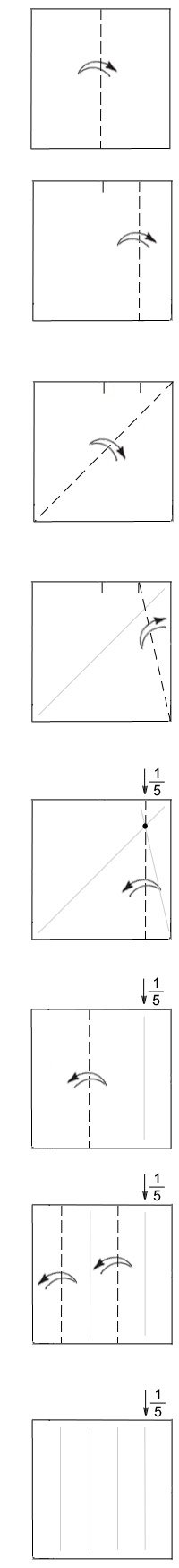# Divide Paper into Fifths: Method 2There are various ways to divide a square sheet of paper into 5 equal segments. The method shown here is fairly easy but leaves 2 crease lines and 2 crease marks along the paper’s edge.#### Divide Paper into Fifths: Method 2

• Fold and unfold a square sheet of paper left to right. You can crease along the entire length of the paper or just make a pinch-crease along the top edge of the paper.

• Fold and unfold the right side of the paper to achieve 1/4 division. Again, you can crease across entire sheet, or just make a pinch.

• Fold and unfold the paper in half along the diagonal. The crease line should be near the 1/4 pinch-mark (fold bottom-right corner up to top-left corner, unfold).

• Looking at the far right side of the paper, fold and unfold the section that is 1/4-wide. Fold along the diagonal so crease-line extends from bottom-right corner of the paper to the pinch-crease at 1/4.

• The location where the two diagonal creases intersect (at the dot) is the division of 4/5 and 1/5. Fold/unfold along this point of intersection to get the first 1/5 division.

• Fold and unfold the section that is 4/5-wide in half to get 2/5 + 2/5 + 1/5 divisions.

• Fold and unfold the 2/5-wide sections in half to achieve five equal divisions.Note: the point where the two lines intersect shows the 1/5 division along the vertical and the horizontal. Thus, a vertical crease or a horizontal crease which passes through the dot gives 1/5 division.